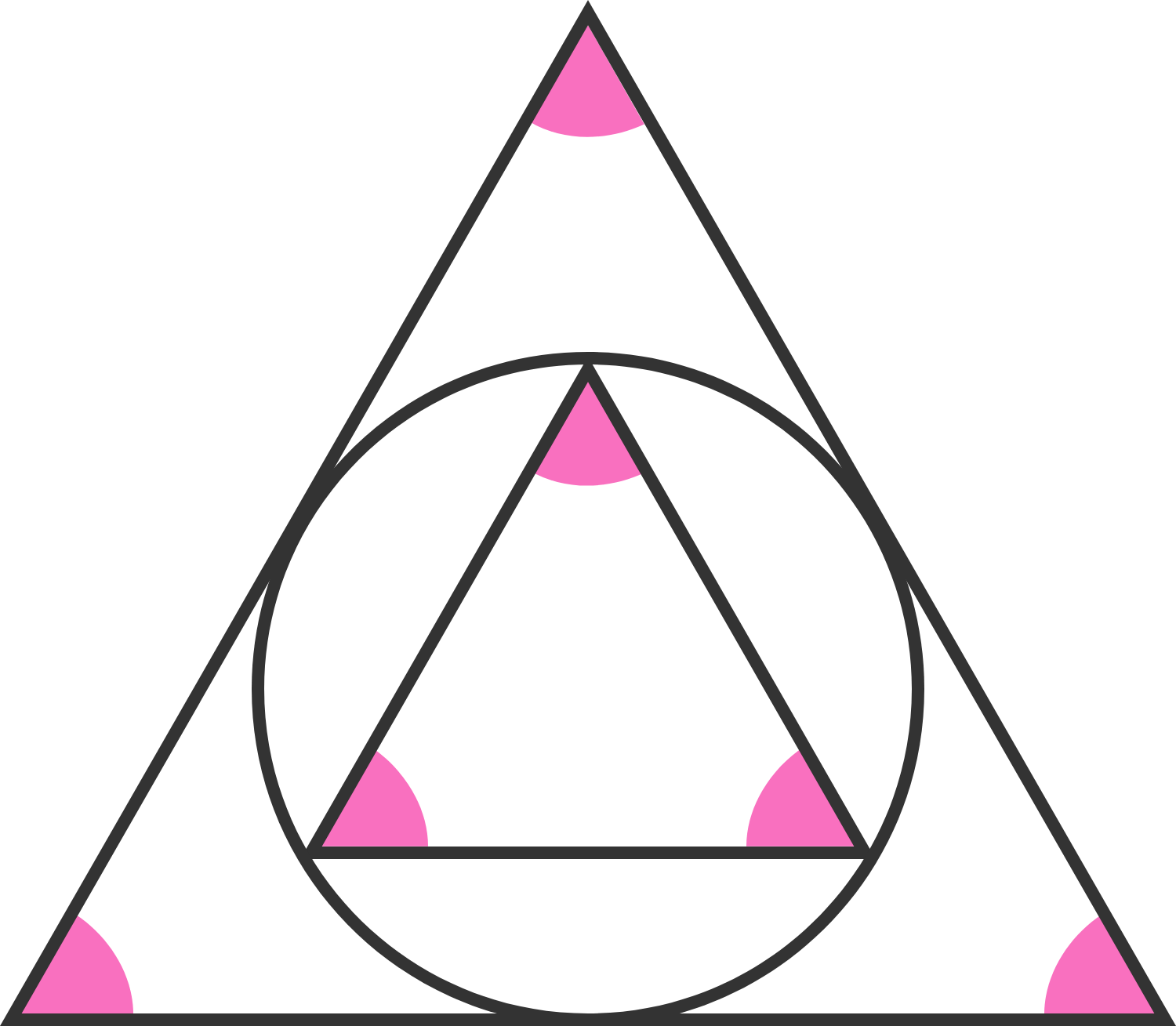# Double Triangles And A Circle

Geometry Level 2There is a circle that touches 3 points of the small triangle. Also it's touching the 3 sides of the bigger triangle. Knowing that both triangles are equilateral triangles, then how many times is the bigger triangle bigger than the smaller one?

×